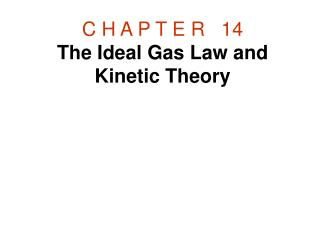DownloadDownload PresentationC H A P T E R   14 The Ideal Gas Law and Kinetic Theory

# C H A P T E R   14 The Ideal Gas Law and Kinetic Theory

Télécharger la présentation## C H A P T E R   14 The Ideal Gas Law and Kinetic Theory

- - - - - - - - - - - - - - - - - - - - - - - - - - - E N D - - - - - - - - - - - - - - - - - - - - - - - - - - -
##### Presentation Transcript

1. C H A P T E R   14The Ideal Gas Law and Kinetic Theory

2. 14.1 The Mole, Avogadro's Number, and Molecular Mass

3. Atomic Mass Unit, U By international agreement, the reference element is chosen to be the most abundant type of carbon, called carbon-12, and its atomic mass is defined to be exactly twelve atomic mass units, or 12 u.

4. Molecular Mass The molecular mass of a molecule is the sum of the atomic masses of its atoms. For instance, hydrogen and oxygen have atomic masses of 1.007 94 u and 15.9994 u, respectively. The molecular mass of a water molecule (H2O) is: 2(1.007 94 u) + 15.9994 u = 18.0153 u.

5. Avogadro's NumberNA The number of atoms per mole is known as Avogadro's numberNA, after the Italian scientist Amedeo Avogadro (1776–1856):

6. Number of Moles, n The number of moles n contained in any sample is the number of particles N in the sample divided by the number of particles per moleNA (Avogadro's number): The number of moles contained in a sample can also be found from its mass.

7. 14.2 The Ideal Gas Law An ideal gas is an idealized model for real gases that have sufficiently low densities.

8. The Ideal Gas Law An ideal gas is an idealized model for real gases that have sufficiently low densities. The condition of low density means that the molecules of the gas are so far apart that they do not interact (except during collisions that are effectively elastic).

9. The Ideal Gas Law An ideal gas is an idealized model for real gases that have sufficiently low densities. The condition of low density means that the molecules of the gas are so far apart that they do not interact (except during collisions that are effectively elastic). The ideal gas law expresses the relationship between the absolute pressure (P), the Kelvintemperature (T), the volume (V), and the number of moles (n) of the gas. Where R is the universal gas constant. R = 8.31 J/(mol · K).

10. The Ideal Gas Law The constant term R/NA is referred to as Boltzmann's constant, in honor of the Austrian physicist Ludwig Boltzmann (1844–1906), and is represented by the symbol k: PV = NkT

11. 14.3 Kinetic Theory of Gases

12. Kinetic Theory of Gases The pressure that a gas exerts is caused by the impact of its molecules on the walls of the container.

13. Kinetic Theory of Gases The pressure that a gas exerts is caused by the impact of its molecules on the walls of the container. It can be shown that the average translational kinetic energy of a molecule of an ideal gas is given by, where k is Boltzmann's constant and T is the Kelvin temperature.

14. Derivation of, Consider a gas molecule colliding elastically with the right wall of the container and rebounding from it.

15. The force on the molecule is obtained using Newton’s second law as follows,

16. The force on one of the molecule, According to Newton's law of action–reaction, the force on the wall is equal in magnitude to this value, but oppositely directed. The force exerted on the wall by one molecule,

17. If N is the total number of molecules, since these particles move randomly in three dimensions, one-third of them on the average strike the right wall. Therefore, the total force is: Vrms = root-mean-square velocity.

18. Pressure is force per unit area, so the pressure P acting on a wall of area L2 is

19. Pressure is force per unit area, so the pressure P acting on a wall of area L2 is Since the volume of the box is V = L3, the equation above can be written as,

20. PV = NkT

21. EXAMPLE 6 The Speed of Molecules in Air Air is primarily a mixture of nitrogen N2 (molecular mass = 28.0 u) and oxygen O2 (molecular mass = 32.0 u). Assume that each behaves as an ideal gas and determine the rms speed of the nitrogen and oxygen molecules when the temperature of the air is 293 K.

22. The Internal Energy of a Monatomic Ideal Gas